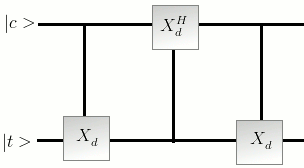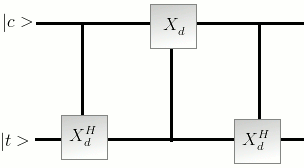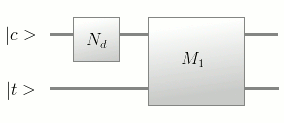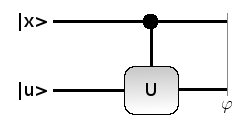Thursday, April 23, 2009

Qudit Swap Gate

We have seen that the contolled-X (C-NOT) operation in d-state systems acts as

Then the Controlled - operation acts as

Using these operators we can make the Qudit Swap Gate.

Now consider the following gates
Call this as Gate M1.Call this as Gate M2Now we can see that operation M1 is

And operation M2 is

Also

So

Now by adding an operation at the end we can form the Qudit swap gates as shownSince the inverse of Swap operation is also Swap operation we have the following implementations alsoTuesday, April 21, 2009

Controlled-U Qudit gate

Consider a Qudit Controlled-U GateLet the Qudit system under consideration have as the d-canonical states and let us consider the ring be the ring of integers from zero to d-1.

Now the controlled-U operation is defined for a qudit sytem as follows

where

and

Using this we see that the qudit version of Controlled NOT gate becomes

So this is in accordance with the d=2 where where had the CNOT operation doing

So the generalized CNOT operation is

Monday, April 20, 2009

Qudit gates

Here we move in to generalize the basic Qubit gates to Qudit gates.

We take some common gates like

Hadamard gate : Hadamard gate is used widely in Qubit literature. Hadamard gate is given by

This we have noticed that is the . Hence we take the as the generalized Qudit gate for the Hadamard gate.

NOT Gate : For a Qubit system with canonical states the not gate acts on a possible state as

We generalize this gate to its Qudit counterpart as where is a canonical qudit state and the operation + is the ring operation over ."1" is the additive identity of

Now suppose then we have

Like wise we get

Tuesday, April 14, 2009

Properties of QFT

Being similar to the DFT matrix, QFT matrixhas all the properties of DFT matrix.

Some properties are listed below

• 1), means is unitary matrix , like every other Quantum Operator.

• 2)• 3)SoNow we focus on the powers of.
We haveWhen m+n = 0(mod d)we haveon other cases the summation is is zero.So we have theletSo we have .

Now (this is easy to prove). then

So we have the following results.

Note that so they commute.

Now let us consider a qudit system with d- cannonical states , Consider the quantum Operator acting on any canonical state , we have

Consider the ring of integers with normal addition and multiplication being the ring operations. Then we can view the operator as

where is a canonical state, where negation is the group negation operation. This is equivalent to time reversal in the DFT case.

Simplest matrices are given below.